# Check Dates in chronological order

Assume you have a date list that has different date formats, as seen in the accompanying picture. In this instance, Excel’s Sort function will fail to sort them appropriately. However, you may convert all various date formats to a particular format, such as mm/dd/yyy, and then arrange them chronologically. This post will demonstrate how to sort dates in chronological order and how to check if a range of dates are in chronological order.

## Explanation

This is an excellent illustration of how the SUMPRODUCT function may be used to overcome the inability of the COUNTIF or COUNTIFS functions to operate. In this scenario, the purpose is to verify that all dates within a certain range are in chronological order and to display a “YES” value only when dates are in chronological order.

The argument is pretty straightforward, although maybe not apparent. Rather of ensuring that all dates are bigger than the previous one, we determine if any prior date is greater than the next. If we discover even one, the dates will be out of sequence. They are if we discover none (zero).

At its heart, this formula uses the SUMPRODUCT function to determine the number of dates in A1:C1 that are larger than or equal to B1:D1:

`=SUMPRODUCT(--(A1:C1>=B1:D1))`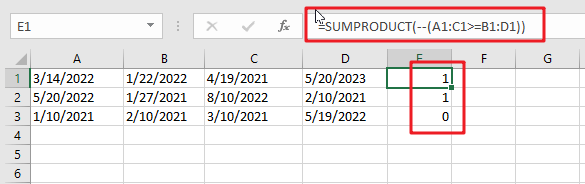Due to the fact that we are employing the larger than or equal to (>=) operator, successive duplicate dates will be disallowed.

This is a two-range operation with 4 dates in each range. As a consequence, an array of TRUE and FALSE values is created as follows: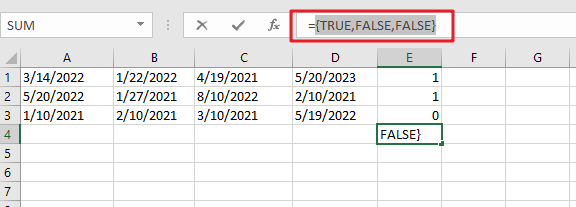You can use the following formula based on the IF function, the SUMPRODUCT function, type:

`=IF(SUMPRODUCT(--(A1:C1>=B1:D1)) =0,”YES”,””)`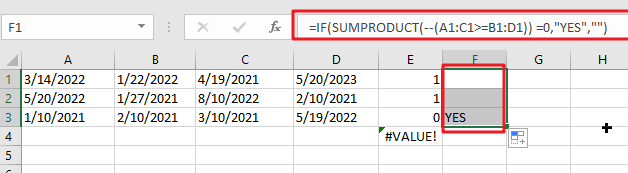In Excel 365, the SORT function offers a convenient alternate solution:

`=IF(SUM(--(A1:D1<>SORT(A1:D1,1,1,1)))=0,"YES","")`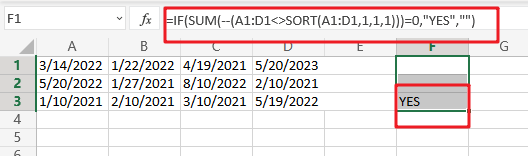The dates in A1:D1 are compared to the same dates following SORT sorting. As with the original algorithm, we are counting any instance when a date is not same (i.e. any date moved by SORT). If there are no dates that vary, a “YES” String Value is returned.

In contrast to the original formula, the SORT version does not check for blank (empty) fields or duplicate dates automatically. The following version has an extra check for blank cells:

`=IF(SUM(((A1:D1<>SORT(A1:D1,1,1,1)))+( A1:D1=""))=0,"YES","")`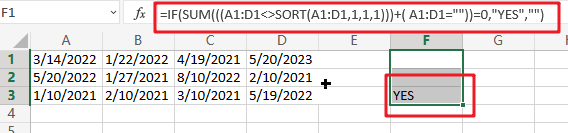In Boolean Algebra, the addition (+) operator behaves similarly to the OR logic. Because the math operation converts TRUE and FALSE values to 1s and 0s automatically, we no longer need the double negative (–).

Note: we use SUM rather than SUMPRODUCT here since Excel 365’s dynamic array support enables SUM to perform array operations natively, without the need for control + shift + enter.

### Related Functions

• Excel SUM function
The Excel SUM function will adds all numbers in a range of cells and returns the sum of these values. You can add individual values, cell references or ranges in excel.The syntax of the SUM function is as below:= SUM(number1,[number2],…)…
• Excel SUMPRODUCT function
The Excel SUMPRODUCT function multiplies corresponding components in the given one or more arrays or ranges, and returns the sum of those products.The syntax of the SUMPRODUCT function is as below:= SUMPRODUCT (array1,[array2],…)…
• Excel COUNTIF function
The Excel COUNTIF function will count the number of cells in a range that meet a given criteria. This function can be used to count the different kinds of cells with number, date, text values, blank, non-blanks, or containing specific characters.etc.= COUNTIF (range, criteria)…
• Excel IF function
The Excel IF function perform a logical test to return one value if the condition is TRUE and return another value if the condition is FALSE. The IF function is a build-in function in Microsoft Excel and it is categorized as a Logical Function.The syntax of the IF function is as below:= IF (condition, [true_value], [false_value])….
• Excel Sort function
The SORT function in Excel sorts the contents of an array or range alphabetically or numerically by columns or rows.The syntax:=SORT(array, [sort index],) …
• Excel COUNTIFS function
The Excel COUNTIFS function returns the count of cells in a range that meet one or more criteria. The syntax of the COUNTIFS function is as below:= COUNTIFS(criteria_range1, criteria1, [criteria_range2, criteria2]…)…